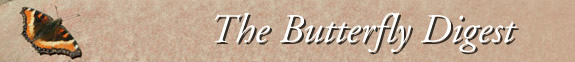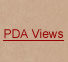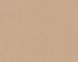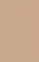Jump to : Today September 29, 2023 September 28, 2023 September 27, 2023 September 26, 2023 September 25, 2023 September 24, 2023 September 23, 2023 September 22, 2023 September 21, 2023 September 20, 2023 September 19, 2023 September 18, 2023 September 17, 2023 September 16, 2023 September 15, 2023 September 14, 2023 September 13, 2023 September 12, 2023 September 11, 2023 September 10, 2023 September 9, 2023 September 8, 2023 September 7, 2023 September 6, 2023 September 5, 2023 September 4, 2023 September 3, 2023 September 2, 2023 September 1, 2023 August 31, 2023     |    View All Lists    |    FAQ
To answer Richard's questions at the bottom:

I have been entering a lot of butterfly records onto m=y Excel file this morning, including a few from Will Stuart in the Sandhill=s Game Land (one list on the same Sept. 12 from Scotland County!), as well =as farther east from Alligator River refuge, the Wilmington area, and the P=iedmont.

Palamedes Swallowtail -- no shortage and =seemingly in normal numbers.=C2=A0 Will had 12 elsewhere in Scotland County= on the game land the same day you were there.=C2=A0 Counts of over 50 in t=he lower Coastal Plain have been routine.

Clou=dless Sulphur -- Will had 15 on the same day down there, and they are commo=n now in the Coastal Plain and Piedmont.=C2=A0 I WILL say, however, they ar=e not as abundant as they often are in late summer, from a few years ago.==C2=A0 So, down in numbers from a handful of years ago, but still should ha=ve been seen in double digits there.=C2=A0 Unlucky?

Sleepy Orange -- About the same as with Cloudless Sulphur status; Will ha=d 18 there the same day.=C2=A0 They are very widespread and common now acro=ss NC.=C2=A0 Maybe they just don't like you?!

=Little Yellow -- Will had 2 of them on the 12th.=C2=A0 Most trips to the Sa=ndhills and southern Coastal Plain are running into a few, as well as in th=e Piedmont and parts of the mountains, but essentially all reports are of 1=-few of them.=C2=A0 Certainly not a good northbound flight for them again t=his year.=C2=A0 So, down a bit, but surprised you didn't see at least o=ne.

Then again, you DID see 2 Byssus, 3 Yehl, =and 7 Dotted skippers, so ... a good tradeoff, I'd say!

<=/div>
Harry LeGrand

On Thu, Sep 14, 2023 at 4:2=2=E2=80=AFPM Richard Stickney <">=> wrote:

Hi all,

=C2=A0

I hit a couple of good spots in the Sandhills Game L=and on Tuesday. I had similar results to some others who have been there la=tely with a few observations.

=C2=A0

Scotland County, Hoffman Road not far from Broadacre=s Lake, in a nice stand of Slender Ironweed:

E. Tiger ST =E2=80=93 5

Palamedes ST =E2=80=93 3 very low

Spicebush ST =E2=80=93 3

Cloudless Sulphur =E2=80=93 1 very low, and no Sleep=y Oranges or Little Yellows

Gray Hairstreak =E2=80=93 2

Red-banded HS =E2=80=93 1

Gulf Fritillary =E2=80=93 1 but no Variegated, which= I=E2=80=99ve always seen

Buckeye =E2=80=93 5

Long-tailed Skipper =E2=80=93 1

Zarucco Duskywing =E2=80=93 2

Byssus Skipper =E2=80=93 1

Fiery Skipper =E2=80=93 2

Dotted Skipper =E2=80=93 7 mostly worn, photos on Fl=ickr

=C2=A0

Richmond County, Derby Road at Naked Creek, lots of =Joe-pye and NY Ironweed:

ETS =E2=80=93 1

Spicebush ST =E2=80=93 1

Summer Azure =E2=80=93 1

Pearl Crescent =E2=80=93 1

Carolina/Intricate Satyr =E2=80=93 3

Silver-spotted Skipper =E2=80=93 1

Dun Skipper =E2=80=93 2

Byssus Skipper =E2=80=93 1

Fiery Skipper =E2=80=93 2

Little Glassywing =E2=80=93 2

Yehl Skipper =E2=80=93 3

=C2=A0

The absence of many late-season butterflies has been= noticeable everywhere I=E2=80=99ve been this year. Where are the Palamedes= ST, Cloudless Sulphurs, Little Yellows, Sleepy Oranges, Ocola Skippers etc==E2=80=A6=E2=80=A6.?

=C2=A0

Richard Stickney

NC Museum of Life & Science

www.flickr.com/photos/rstickney

=C2=A0

=C2=A0

--00000000000077f7ea0605a45bdd--